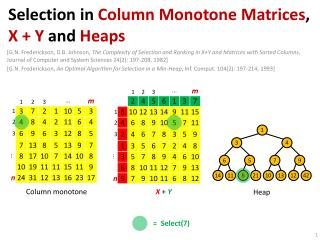Download PresentationSelection in Column Monotone Matrices , X + Y and Heaps

# Selection in Column Monotone Matrices , X + Y and Heaps - PowerPoint PPT Presentation

Selection in Column Monotone Matrices , X + Y and Heaps. 1. [G.N. Frederickson, D.B. Johnson, The Complexity of Selection and Ranking in X+Y and Matrices with Sorted Columns , Journal of Computer and System Sciences 24(2): 197-208, 1982]I am the owner, or an agent authorized to act on behalf of the owner, of the copyrighted work described.
Download Presentation## Selection in Column Monotone Matrices , X + Y and Heaps

Download Policy: Content on the Website is provided to you AS IS for your information and personal use and may not be sold / licensed / shared on other websites without getting consent from its author.While downloading, if for some reason you are not able to download a presentation, the publisher may have deleted the file from their server.

- - - - - - - - - - - - - - - - - - - - - - - - - - E N D - - - - - - - - - - - - - - - - - - - - - - - - - -
Presentation Transcript
1. Selection in Column Monotone Matrices, X + Y and Heaps 1 [G.N. Frederickson, D.B. Johnson, The Complexity of Selection and Ranking in X+Y and Matrices with Sorted Columns, Journal of Computer and System Sciences 24(2): 197-208, 1982] [G.N. Frederickson, An Optimal Algorithm for Selection in a Min-Heap, Inf. Comput. 104(2): 197-214, 1993] 3 4 6 5 7 9 14 11 8 21 10 13 12 42 Column monotone X+ Y Heap = Select(7)

2. Partition (I1,i,I2) jI1 : xj  xijI2 : xi  xj Select(k)  find partitionwith |I1|+1 = k Select(6) Algorithm : BinarysearchusingSelect Time : O(n + n/2 + n/4 + ∙∙∙ + 2 + 1) = O(n) [M. Blum, R.W. Floyd, V. Pratt, R. Rivest and R. Tarjan, Time bounds for selection, J. Comp. Syst. Sci. 7 (1973) 448-461] <p >p T(n) = n + T(n/5) + T(7n/10) = O(n) Weighted-Select(w) Find partition with w - wi ΣjI1wj < w Weighted-Select(18)

3. Selection in Column Monotone Matrices m k m k/2 k m k m k ... k∙logk Selectk Selectk/2 1 2 k k k k n n n n (permute columns) (log kiterations) 3 Result so far... Identified O(k) elements in prefixes of p = min{m,k} columns Time so far... O(m + p + p/2 + p/4 +∙∙∙+ 1) = O(m) weight(x) x Weighted-Select(k)on k+k/2+k/4+∙∙∙+1= O(k) elements 2kcandidatesleft (green + yellow)

4. Selection in Column Monotone Matrices Select(k,N,p) assumek  N/2 (N = total length of the p lists)  2N  0.086∙N Elliminate  = 1- 1/2 = 0.29 ∙length   x x x recurse • (1-)2N = N/2 • i.e. k’th smallest not elliminated N (1-)N Weighted-Select(N) Weight of element = length of list Result so far... Identified O(k) elements in prefixes of p = min{m,k} columns Time so far... O(m + p + p/2 + p/4 +∙∙∙+ 1) = O(m) • N = O(p)  Select(k) • k< N/2  symmetricwithreverseorder • T(N) = p + T( (1-2)∙N) =O(p∙log (N/p)) Total time O(m+p∙log(k/p)), p = min { k, m} k = O(m) : O(m) k = (m) : O(m∙log(k/m))

5. Selection in X + Y ̶ reuse column monotone algorithm ? n  m m k m k/2 k m k m k ... k∙logk Selectk Selectk/2 1 2 k k k k n n n n (permute columns) (log kiterations) X works Time O(m) 21 3 22 weight(x) < < < < < < x 23 Weighted-Select(k)onk+k/2+k/4+∙∙∙+1= O(k) elements 2kcandidatesleft Semi-sorting 2-powers Time n+n/2+n/4+∙∙∙+1 = O(n)

6. Selection in X + Y ̶ reuse column monotone algorithm ? Select(k,N,p) assumek  N/2 (N = total length of the p lists)  = 1/4 Total time O(m+p∙log(k/p)), p = min { k, m} k = O(m) : O(m) k = (m) : O(m+k∙log(k/m)) ∙length (the same) X 21 < < < < < < 22 Eachiterationrefinesemi-sorting  O(n) per level 23 Canapproximate sample by a closeenoughsemi-sorted element Semi-sorting 2-powers Time n+n/2+n/4+∙∙∙+1 = O(n)

7. [F93] considersadditionally... • How to computerank(x) in column monotone and X+Y matrices in O(m+p∙log(k/p)), p = min {k, m} • Provesthat the boundsareoptimal

8. Selection in a BinaryHeap • [G.N. Frederickson, An Optimal Algorithm for Selection in a Min-Heap, Inf. Comput. 104(2): 197-214, 1993] front • k x DeleteMin O(k∙logn) • k x DeleteMinfront  O(k∙logk) k∙logk  k∙loglogk  k∙logloglogk  k∙3log* k k∙2log* k  k k smallest in sortedorder Ω(k∙logk)lowerbound only the kthsmallest  Ω(k)lowerbound • clans of size log k • auxiliary heap of clan representatives (max) • ExtractMin representative & construct 2 clans • k/log k’thExtractMin representative x has rank k..2k • select(k) on all elements  x • Total time O(k∙loglogk + k/log k∙logk + k) 1 clan 3 4 max defined from poor relatives of Ci 6 5 7 9 Ci defined from offsprings of Ci 14 11 8 21 10 13 12 42 poor relatives Cj Cj clan construction auxiliary heap min selection offspringsCj